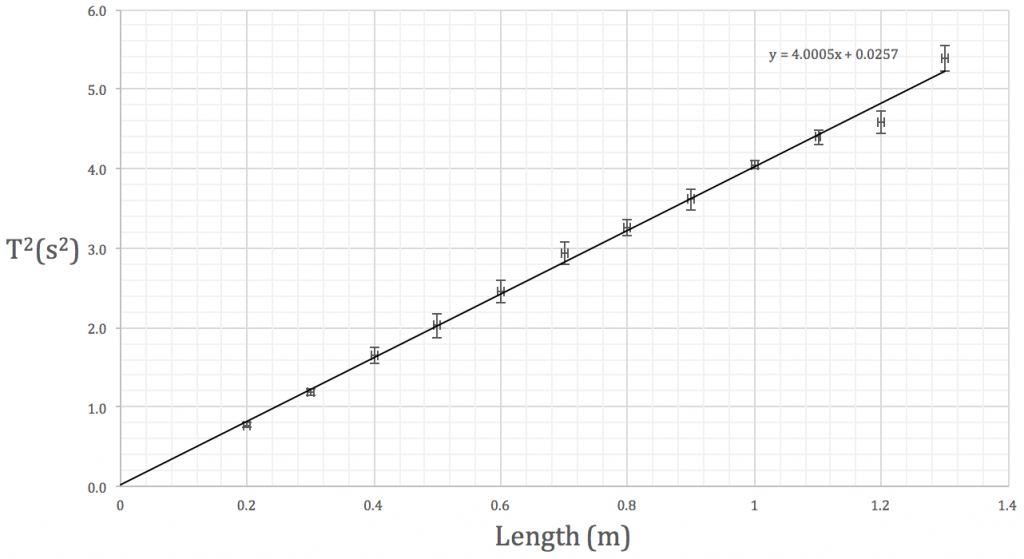# using Excel’s LINEST function

The period T of a simple pendulum can be calculated using$T=2 \pi \displaystyle \sqrt{l \over g}$

where l is the pendulum length and g is the gravitational field strength.

Using a single value of length and period, we can determine the acceleration due to gravity.  However, it would be better experimental practise to vary the length of the pendulum and plot a graph of$T^2$ against length, determining g from the gradient of the line of best fit.We’re going to spend the next three periods analysing your simple pendulum data in the library.  The attached pdf will walk you through the steps.  It would be better if you used your own results but I’ve put some sample data on the first page if you’ve forgotten to bring yours.

This site uses Akismet to reduce spam. Learn how your comment data is processed.